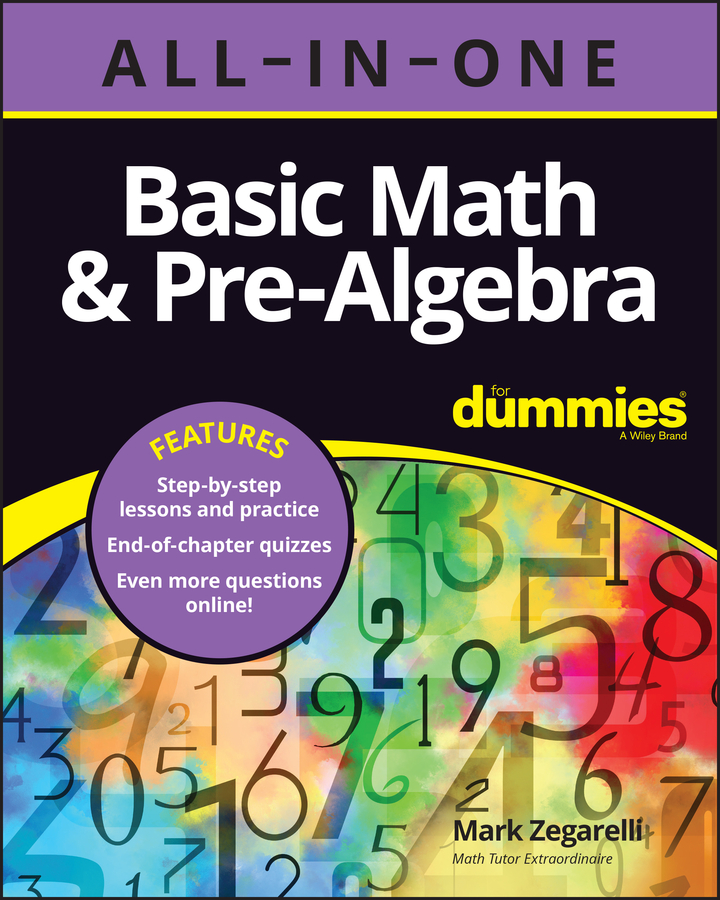##### Basic Math & Pre-Algebra All-in-One For Dummies (+ Chapter Quizzes Online)If you know how to round numbers, you can use this skill in estimating values. Estimating saves you time by allowing you to avoid complicated computations and still get an approximate answer to a problem.

When you get an approximate answer, you don’t use an equal sign; instead, you use this wavy symbol, which means is approximately equal to:

Suppose you want to add these numbers: 722 + 506 + 383 + 1,279 + 91 + 811. This computation is tedious, and you may make a mistake. But you can make the addition easier by first rounding all the numbers to the nearest hundred and then adding:

700 + 500 + 400 + 1,300 + 100 + 800 = 3,800

The approximate answer is 3,800. This answer isn’t very far off from the exact answer, which is 3,792.

Similarly, suppose you want to multiply 879 618. Again, this computation doesn’t look like a lot of fun. Instead, you can round these numbers to the nearest hundred:

900 600 = 540,000

This time, the approximate answer is 540,000, and the exact answer is 543,222. Again, not a bad estimate.

Estimating is useful, but it can also lead to results that aren’t very close to the right answer. You’re most likely to arrive at a bad estimate when you

• Round numbers that are close to the middle of the range

• Round too many numbers in the same direction (either up or down)

• Multiply or divide rounded numbers

For example, suppose you want to multiply 349 243. You start by rounding both of these numbers:

300 200 = 60,000

Your estimate is 60,000, but the actual answer is 84,807 — not a very good approximation. What happened? First, notice that 349 is very close to the middle of the range between 300 and 400. And 247 is close to the middle of the range between 200 and 300. So when you rounded these numbers, you changed their values a lot. Second, you rounded both numbers down to lower values. That’s why the estimate ended up so much lower than the real answer. Third, you multiplied. In general, multiplying and dividing throw off estimates more than addition and subtraction do.